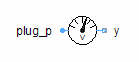Tellinen Permanent Magnet - MapleSim Help

Tellinen Permanent Magnet

Permanent magnet based on the Tellinen hysteresis modelDescription The Tellinen Permanent Magnet component is a flux tube element of fixed length and cross-sectional area that models the hard magnetic hysteresis of permanent magnets. The model is similar to Tellinen Hard but has an initial magnetization preset of -100% and an adapted icon for better readability of the diagram.Equations $\mathrm{\Phi }={\mathrm{\Phi }}_{p}=-{\mathrm{\Phi }}_{n}=BA$ ${V}_{m}={V}_{{m}_{p}}-{V}_{{m}_{n}}$ $H=\frac{{V}_{m}}{\ell }={H}_{\mathrm{stat}}+{H}_{\mathrm{eddy}}$ ${H}_{\mathrm{eddy}}=\left\{\begin{array}{cc}\frac{\mathrm{\sigma }{d}^{2}}{12}\frac{\mathrm{dB}}{\mathrm{dt}}& \mathrm{Include eddy currents}\\ 0& \mathrm{otherwise}\end{array}$ ${H}_{0}=\frac{1}{2}\mathrm{log}\left(\frac{1+{\mathrm{\mu }}_{0}\frac{{H}_{c}}{{B}_{r}}}{1-{\mathrm{\mu }}_{0}\frac{{H}_{c}}{{B}_{r}}}\right)+M{H}_{c}$ ${\mathrm{hyst}}_{F}={J}_{s}\mathrm{tanh}\left(\frac{{H}_{\mathrm{stat}}M+{H}_{0}}{{H}_{\mathrm{unit}}}\right)+{\mathrm{\mu }}_{0}{H}_{\mathrm{stat}}+\frac{1}{2}\mathrm{eps}$ ${\mathrm{hyst}}_{R}={J}_{s}\mathrm{tanh}\left(\frac{{H}_{\mathrm{stat}}M-{H}_{0}}{{H}_{\mathrm{unit}}}\right)+{\mathrm{\mu }}_{0}{H}_{\mathrm{stat}}-\frac{1}{2}\mathrm{eps}$ $\left\{\begin{array}{cc}\left\{\mathrm{dHyst}=0,k=\frac{1}{100}\right\}& \mathrm{initial}\\ \left\{\mathrm{dHyst}=\frac{d}{\mathrm{dt}}\left(-{H}_{\mathrm{stat}}{\mathrm{\mu }}_{0}+{\mathrm{hyst}}_{R}\right),k=\mathrm{max}\left(\frac{1}{100},\frac{{\mathrm{hyst}}_{F}-B}{\Delta \mathrm{hyst}}\right)\right\}& 0<\frac{{\mathrm{dH}}_{\mathrm{stat}}}{\mathrm{dt}}\\ \left\{\mathrm{dHyst}=\frac{d}{\mathrm{dt}}\left(-{H}_{\mathrm{stat}}{\mathrm{\mu }}_{0}+{\mathrm{hyst}}_{F}\right),k=\mathrm{max}\left(\frac{1}{100},\frac{B-{\mathrm{hyst}}_{R}}{\Delta \mathrm{hyst}}\right)\right\}& \mathrm{otherwise}\end{array}$ $\mathrm{LossPower}={\mathrm{LossPower}}_{\mathrm{stat}}+{\mathrm{LossPower}}_{\mathrm{eddy}}$ ${\mathrm{LossPower}}_{\mathrm{eddy}}={H}_{\mathrm{eddy}}\frac{\mathrm{dB}}{\mathrm{dt}}V$ ${\mathrm{LossPower}}_{\mathrm{stat}}={H}_{\mathrm{stat}}\frac{\mathrm{dB}}{\mathrm{dt}}V$ $\Delta \mathrm{hyst}={\mathrm{hyst}}_{F}-{\mathrm{hyst}}_{R}$ $\frac{\mathrm{dB}}{\mathrm{dt}}=k\mathrm{dHyst}+{\mathrm{\mu }}_{0}\frac{d{H}_{\mathrm{stat}}}{\mathrm{dt}}$ $\frac{\mathrm{dMagRel}}{\mathrm{dt}}=0$ ${T}_{\mathrm{hp}}=\left\{\begin{array}{cc}{T}_{\mathrm{heatPort}}& \mathrm{Use Heat Port}\\ T& \mathrm{otherwise}\end{array}$Variables

 Name Units Description Modelica ID $B$ $T$ Magnetic flux density B $\mathrm{dHyst}$ $\frac{T}{s}$ Slope of the limiting hysteresis branch dHyst $H$ $\frac{A}{m}$ Magnetic field strength H ${H}_{\mathrm{eddy}}$ $\frac{A}{m}$ Dynamic (eddy currents) portion of the magnetic field strength Heddy ${H}_{\mathrm{stat}}$ $\frac{A}{m}$ Static (ferromagnetic) portion of the magnetic field strength Hstat $\mathrm{LossPower}$ $W$ Loss power leaving component via HeatPort LossPower ${\mathrm{LossPower}}_{\mathrm{eddy}}$ $W$ Eddy current losses (dynamic hysteresis losses) LossPowerEddy ${\mathrm{LossPower}}_{\mathrm{stat}}$ $W$ Ferromagnetic (static) hysteresis losses LossPowerStat $\mathrm{MagRel}$ $1$ Relative magnetization at initialization (-1..1) MagRel $\mathrm{\Phi }$ $\mathrm{Wb}$ Magnetic flux from port_p to port_n Phi ${T}_{\mathrm{heatPort}}$ $K$ Temperature of HeatPort T_heatPort ${V}_{m}$ $A$ Magnetic potential difference between both ports V_mConnections

 Name Description Modelica ID ${\mathrm{port}}_{p}$ Positive magnetic port port_p ${\mathrm{port}}_{n}$ Negative magnetic port port_n $\mathrm{heatPort}$ heatPortParametersHystersis

 Name Default Units Description Modelica ID ${B}_{r}$ $1.2$ $T$ Remanence Br ${H}_{c}$ $5·{10}^{5}$ $\frac{A}{m}$ Coercitivity Hc $K$ $1$ $1$ ${\mathrm{\mu }}_{0}$ multiplier K $M$ $10\frac{{H}_{\mathrm{unit}}}{{H}_{c}}$ $1$ Slope of tanh function MFixed Geometry

 Name Default Units Description Modelica ID $\ell$ $0.1$ $m$ Length in direction of flux l $A$ $1·{10}^{-4}$ ${m}^{2}$ Area of cross section ALosses And Heat Parameters

 Name Default Units Description Modelica ID Use Heat Port $\mathrm{false}$ True (checked) enables heat port useHeatPort Include Eddy Currents $\mathrm{false}$ True (checked) enables eddy current losses includeEddyCurrents $\mathrm{\sigma }$ $1·{10}^{7}$ $\frac{S}{m}$ Conductivity of core material sigma $d$ $5·{10}^{-4}$ $m$ Thickness of lamination dModelica Standard Library The component described in this topic is from the Modelica Standard Library. To view the original documentation, which includes author and copyright information, click here.